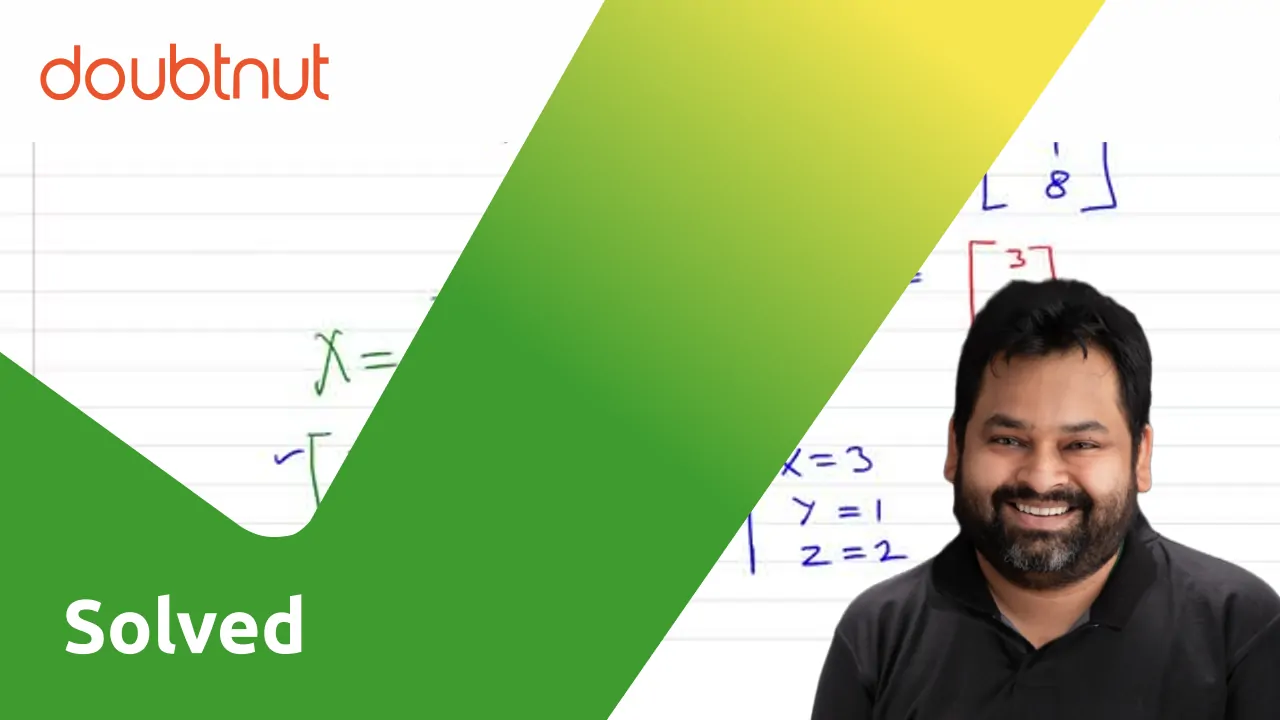Doubtnut App - Get 50 Lakh+ video solutions better than GoogleInstall Now

# Solve by matrix inversion method the following system of equations. x + y + z = 6, x + 2z = 7, 3x + y + z = 12Solution in Bengali

Text Solution
Verified by Experts

## The correct Answer is:x = 1, y = 1 ,z =2

Video Solution